# 1 Tire wear experiment

A fleet manager wishes to compare the wearability of 4 brands of tire: A, B, C, and D. Four cars are available for the experiment and 4 tires of each brand are available, for a total of 16 tires. The idea is to mount 4 tires on each of the 4 cars, ask the driver of each of the cars to drive his/her car for 20,000 miles and then to measure tread loss. We will measure tread loss in mils (0.001 inches). We will designate the 4 cars as cars c1, c2, c3, and c4.

We consider 3 experimental designs.

# 2 Design 1: Brands are randomized to Cars

Naive design.

       Car
c1 c2 c3 c4
-----------
Brand   A  B  C  D
A  B  C  D
A  B  C  D
A  B  C  D
-----------

## 2.1(1 p) What is the obvious flaw in this design?

### 2.1.1 Solution

[answer, 1 sentence describing the problem.]

# 3 Design 2: Completely randomized design (CRD)

Another design that negates the confounding is to use a completely randomized design (CRD). This entails numbering the 16 tires, drawing at random the numbers and assigning tires to cars in a completely random manner. The following table illustrates a possible result of doing this.

       Car
c1 c2 c3 c4    c1 c2 c3 c4
-----------    -----------
Brand   C  A  C  A    12 14 10 13
A  A  D  D    17 13 11  9
D  B  B  B    13 14 14  8
D  C  B  C    11 12 13  9
-----------    -----------

Bring this data in R.

library(tidyverse)

# Plan:
# Read the data values and the treatment labels,
# reshape both into long format and combine.

c1 c2 c3 c4
12 14 10 13
17 13 11  9
13 14 14  8
11 12 13  9

c1 c2 c3 c4
C  A  C  A
A  A  D  D
D  B  B  B
D  C  B  C
", header = TRUE, as.is = TRUE)

d2_dat_long <-
d2_dat %>%
pivot_longer(
cols = everything()
, names_to  = "Car"
, values_to = "Wear"
) %>%
mutate(
Car = factor(Car)
)
d2_trt_long <-
d2_trt %>%
pivot_longer(
cols = everything()
, names_to  = "Car"
, values_to = "Brand"
) %>%
mutate(
Car   = factor(Car)
, Brand = factor(Brand)
)

d2_all <-
bind_cols(
d2_dat_long
, d2_trt_long %>% select(Brand)
#, Brand = d2_trt_long$Brand ) str(d2_all) Classes 'tbl_df', 'tbl' and 'data.frame': 16 obs. of 3 variables:$ Car  : Factor w/ 4 levels "c1","c2","c3",..: 1 2 3 4 1 2 3 4 1 2 ...
$Wear : int 12 14 10 13 17 13 11 9 13 14 ...$ Brand: Factor w/ 4 levels "A","B","C","D": 3 1 3 1 1 1 4 4 4 2 ...

The appropriate analysis for this experiment is the one-way ANOVA.

# Group means
m_d2 <-
d2_all %>%
group_by(Brand) %>%
summarise(
m = mean(Wear)
) %>%
ungroup()

m_d2
# A tibble: 4 x 2
Brand     m
<fct> <dbl>
1 A      14.2
2 B      12.2
3 C      10.8
4 D      11  
# Plot the data using ggplot
library(ggplot2)
p <- ggplot(d2_all, aes(x = Brand, y = Wear))
# plot a reference line for the global mean (assuming no groups)
p <- p + geom_hline(yintercept = mean(d2_all$Wear), colour = "black", linetype = "dashed", size = 0.3, alpha = 0.5) # boxplot, size=.75 to stand out behind CI #p <- p + geom_boxplot(size = 0.75, alpha = 0.5) # points for observed data p <- p + geom_point(aes(shape = Car, colour = Car), position = position_jitter(w = 0.2, h = 0), alpha = 1, size = 2) # diamond at mean for each group p <- p + stat_summary(fun.y = mean, geom = "point", shape = 18, size = 6, colour = "red", alpha = 0.8) # confidence limits based on normal distribution p <- p + stat_summary(fun.data = "mean_cl_normal", geom = "errorbar", width = .2, colour = "red", alpha = 0.8) p <- p + labs(title = "Design 2: Tire Wear") + ylab("Wear (mil)") print(p)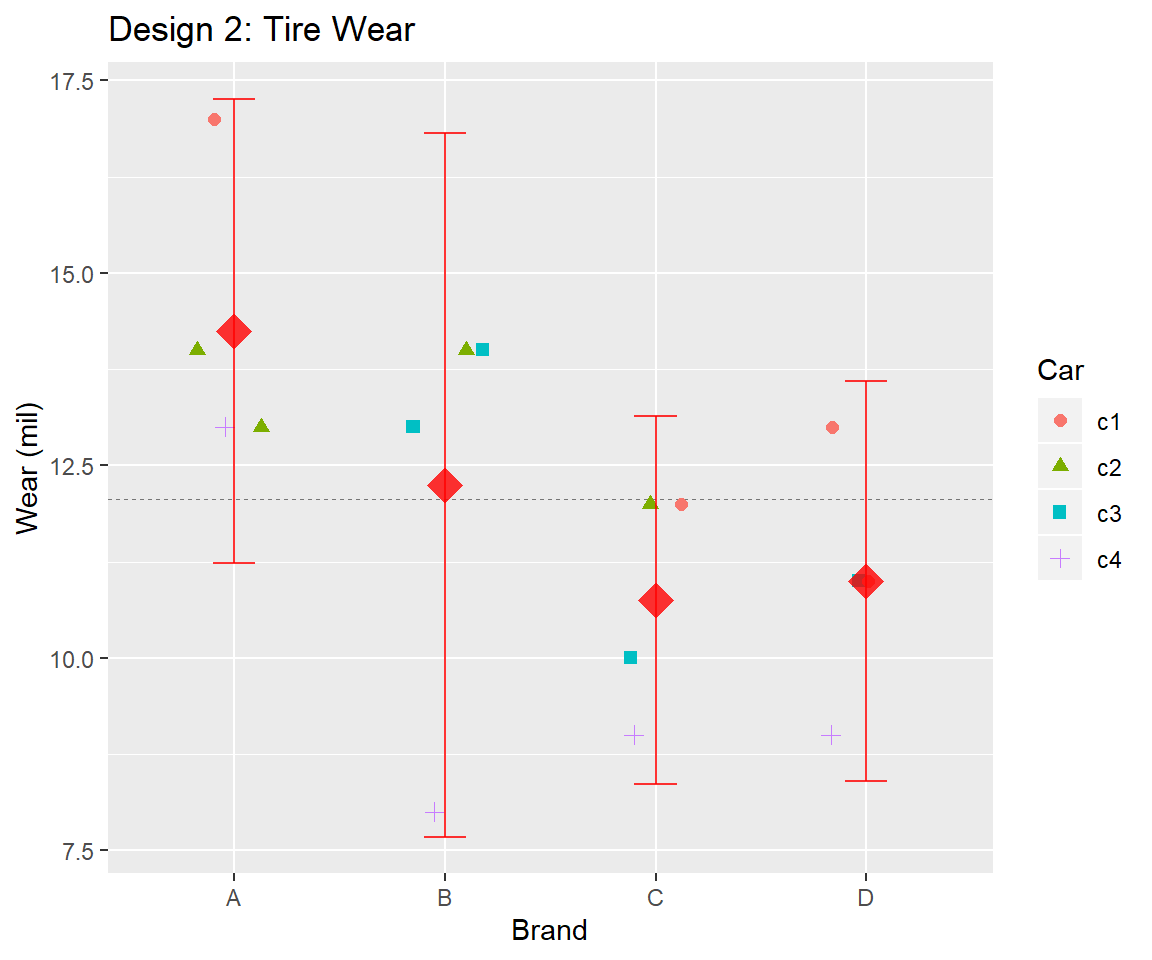## 3.1 Fit model fit_d2 <- lm(Wear ~ Brand, data = d2_all) ## 3.2(1 p) Are the assumptions for the one-way ANOVA met?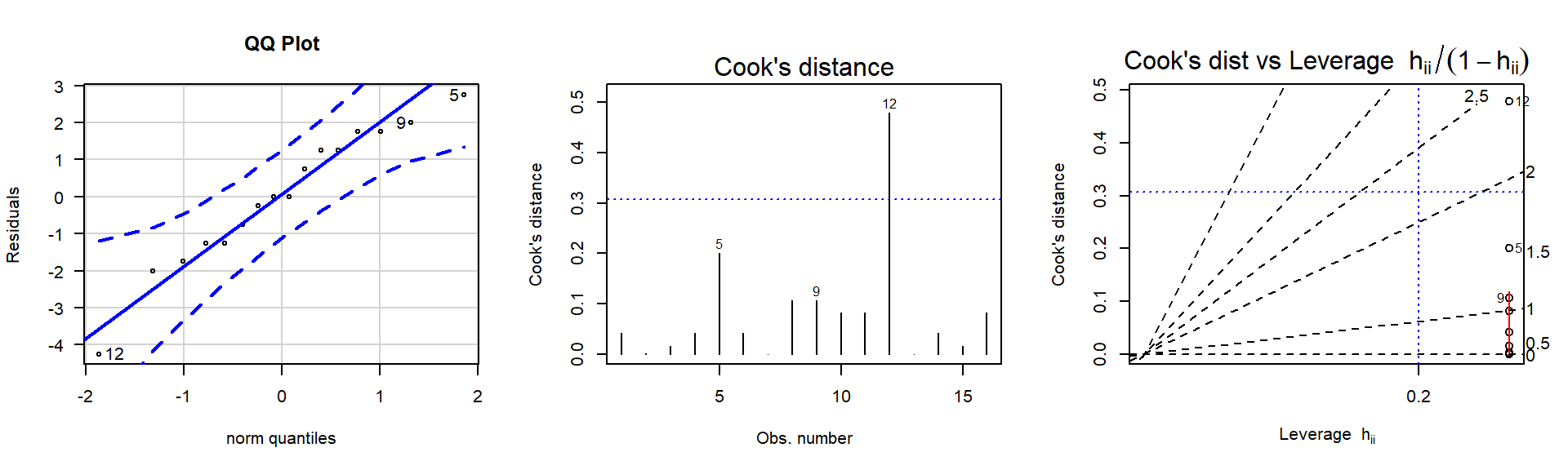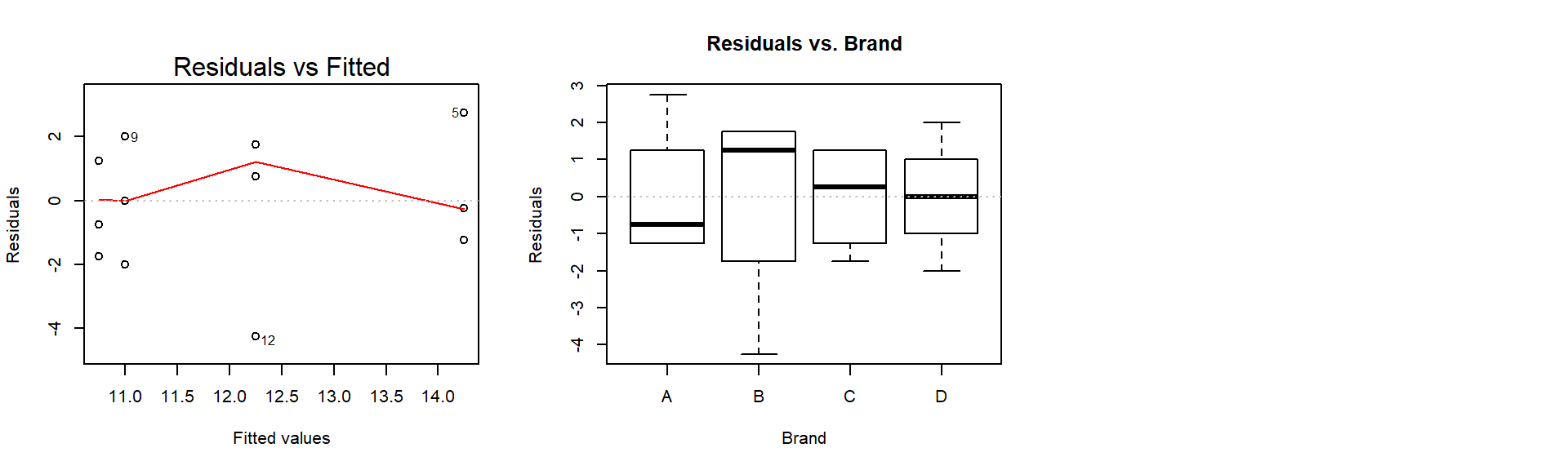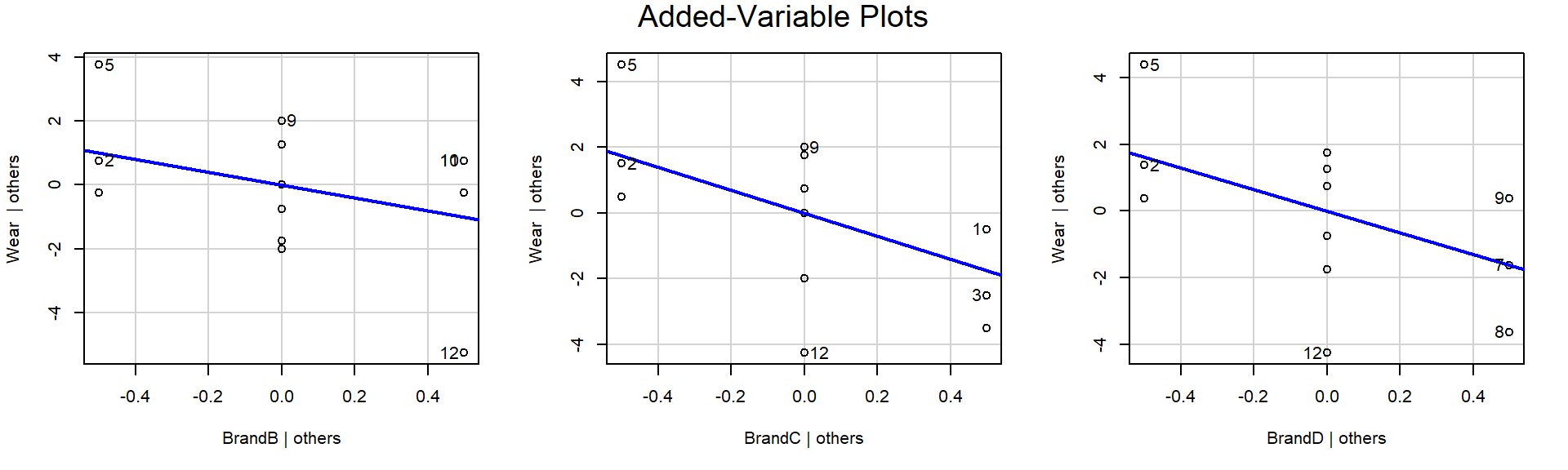Anderson-Darling normality test data: fit_d2$residuals
A = 0.25561, p-value = 0.6783

## 3.3(1 p) Can we infer a difference in mean wear levels between the 4 Brands?

library(car)
Anova(fit_d2, type=3)
Anova Table (Type III tests)

Response: Wear
Sum Sq Df  F value    Pr(>F)
(Intercept) 812.25  1 193.9701 9.051e-09 ***
Brand        30.69  3   2.4428    0.1145
Residuals    50.25 12
---
Signif. codes:  0 '***' 0.001 '**' 0.01 '*' 0.05 '.' 0.1 ' ' 1

# 4 Design 3: Randomized Complete Block Design (RCBD)

In this case, each car tests all four brands. Thus one tire from each brand is selected at random and randomly allocated to the 4 wheels of car c1. Then one tire from each brand is selected and the four are randomly allocated to car c2, and so forth. Here are the results of that design.

       Car
c1 c2 c3 c4    c1 c2 c3 c4
-----------    -----------
Brand   B  D  A  C    14 11 13  9
C  C  B  D    12 12 13  9
A  B  D  B    17 14 11  8
D  A  C  A    13 14 10 13
-----------    -----------

d3_all <- read.table(text="
Car Wear Brand
c1  14   B
c1  12   C
c1  17   A
c1  13   D
c2  11   D
c2  12   C
c2  14   B
c2  14   A
c3  13   A
c3  13   B
c3  11   D
c3  10   C
c4   9   C
c4   9   D
c4   8   B
c4  13   A
mutate(
Car   = factor(Car)
, Brand = factor(Brand)
)

str(d3_all)
'data.frame':   16 obs. of  3 variables:
$Car : Factor w/ 4 levels "c1","c2","c3",..: 1 1 1 1 2 2 2 2 3 3 ...$ Wear : int  14 12 17 13 11 12 14 14 13 13 ...
$Brand: Factor w/ 4 levels "A","B","C","D": 2 3 1 4 4 3 2 1 1 2 ... Means and plots by Brand and by Car. # Group means m_d3_b <- d3_all %>% group_by(Brand) %>% summarise( m = mean(Wear) ) %>% ungroup() m_d3_c <- d3_all %>% group_by(Car) %>% summarise( m = mean(Wear) ) %>% ungroup() m_d3_b # A tibble: 4 x 2 Brand m <fct> <dbl> 1 A 14.2 2 B 12.2 3 C 10.8 4 D 11  m_d3_c # A tibble: 4 x 2 Car m <fct> <dbl> 1 c1 14 2 c2 12.8 3 c3 11.8 4 c4 9.75 par(mfrow=c(1,2)) boxplot(split(d3_all$Wear, d3_all$Brand)) boxplot(split(d3_all$Wear, d3_all$Car))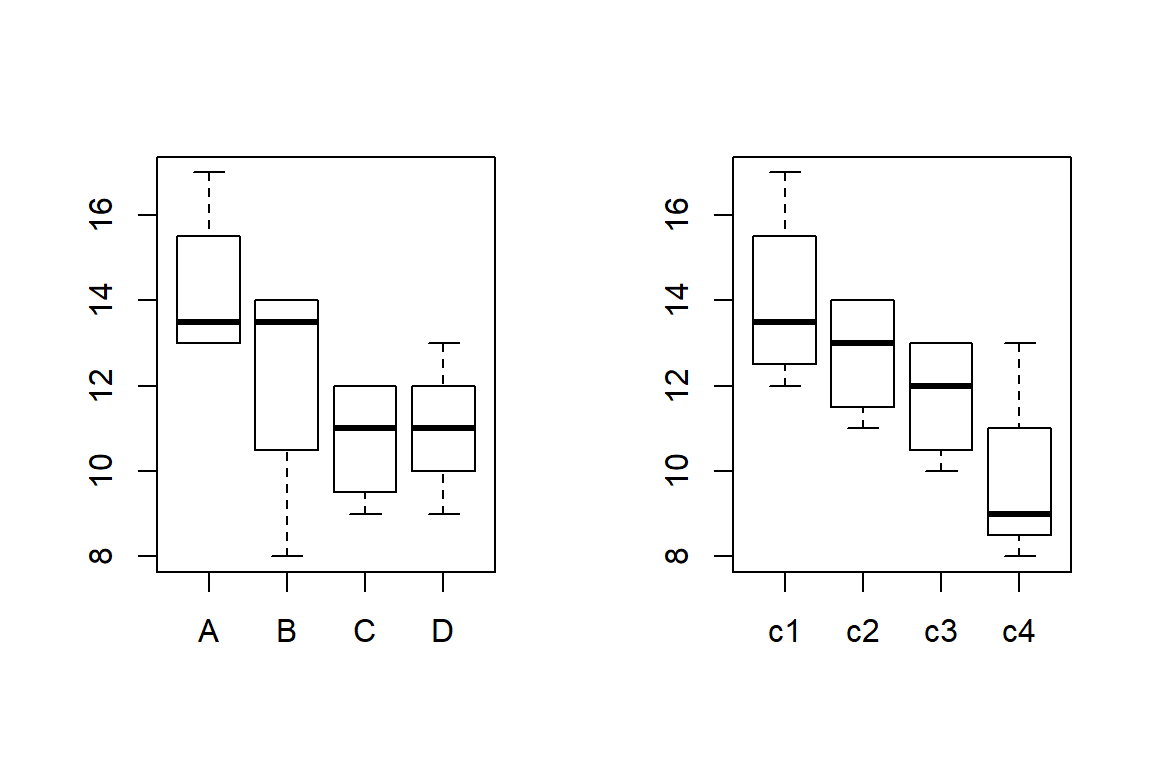par(mfrow=c(1,1)) # Plot the data using ggplot library(ggplot2) p <- ggplot(d3_all, aes(x = Brand, y = Wear)) # plot a reference line for the global mean (assuming no groups) p <- p + geom_hline(yintercept = mean(d3_all$Wear),
colour = "black", linetype = "dashed", size = 0.3, alpha = 0.5)
# boxplot, size=.75 to stand out behind CI
#p <- p + geom_boxplot(size = 0.75, alpha = 0.5)
# points for observed data
p <- p + geom_point(aes(shape = Car, colour = Car), position = position_jitter(w = 0.2, h = 0), alpha = 1, size = 2)
# colored line for each Care
p <- p + geom_line(aes(group = Car, colour = Car), alpha = 0.5)
# diamond at mean for each group
p <- p + stat_summary(fun.y = mean, geom = "point", shape = 18, size = 6,
colour = "red", alpha = 0.8)
# confidence limits based on normal distribution
p <- p + stat_summary(fun.data = "mean_cl_normal", geom = "errorbar",
width = .2, colour = "red", alpha = 0.8)
p <- p + labs(title = "Design 3: Tire Wear") + ylab("Wear (mil)")
print(p)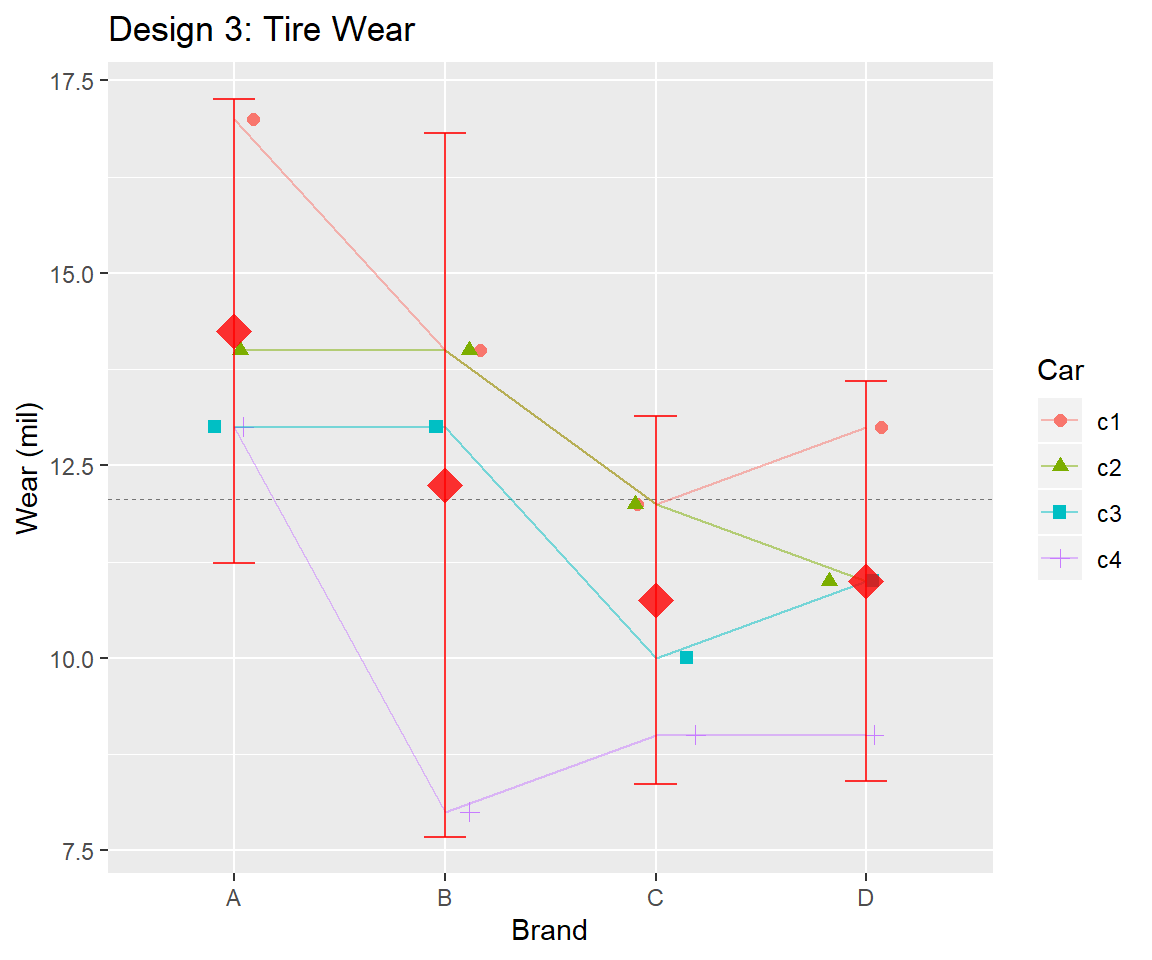## 4.1(2 p) Briefly, what relationships are there between Wear and Brand or Car?

Refer to the numerical and graphical summaries above.

## 4.2 Fit model

fit_d3 <- lm(Wear ~ Brand + Car, data = d3_all)

## 4.3(1 p) Are the assumptions for the RCBD met?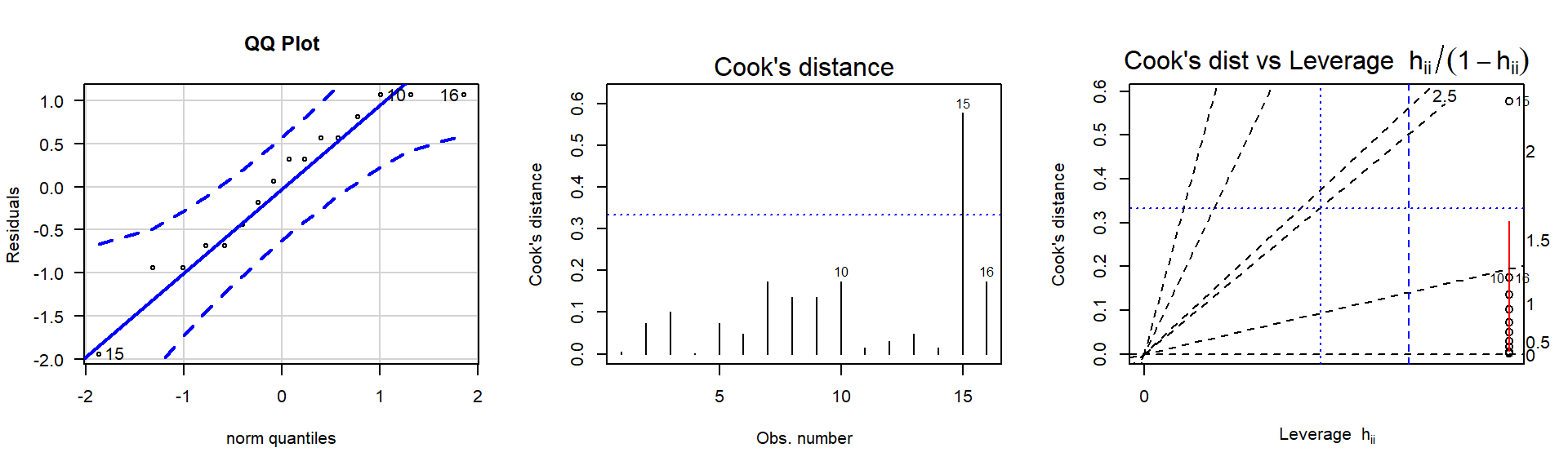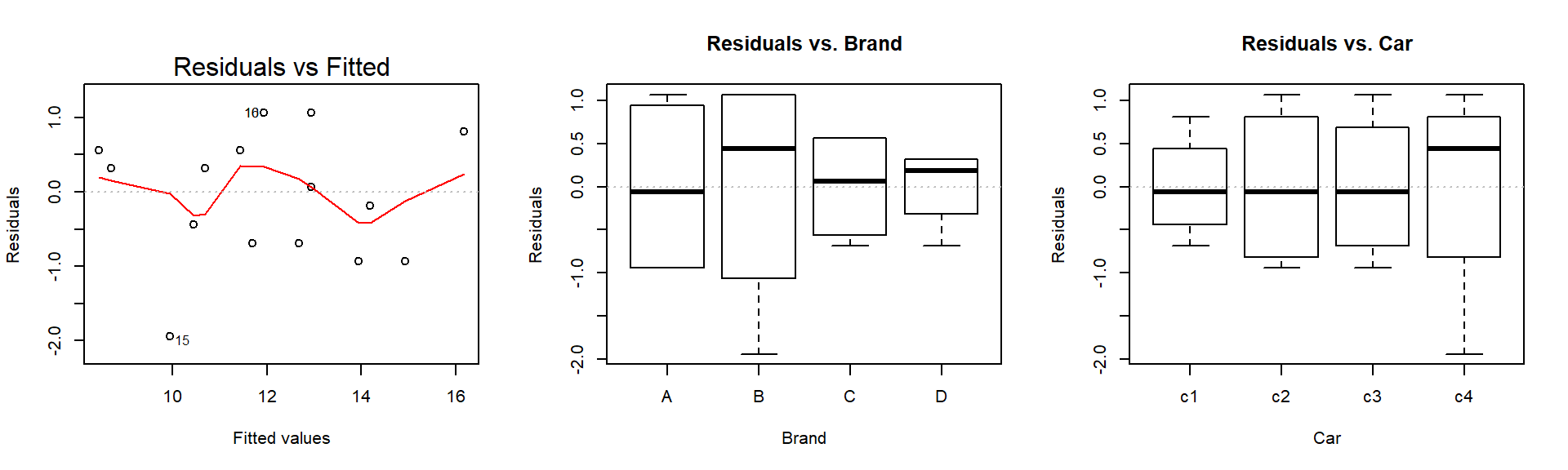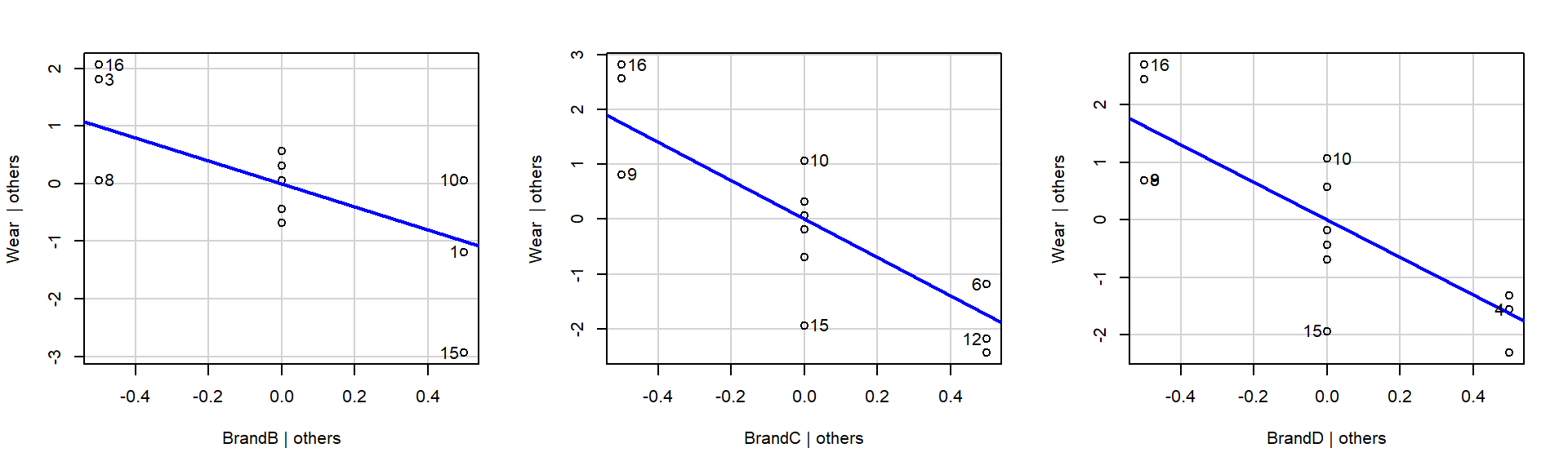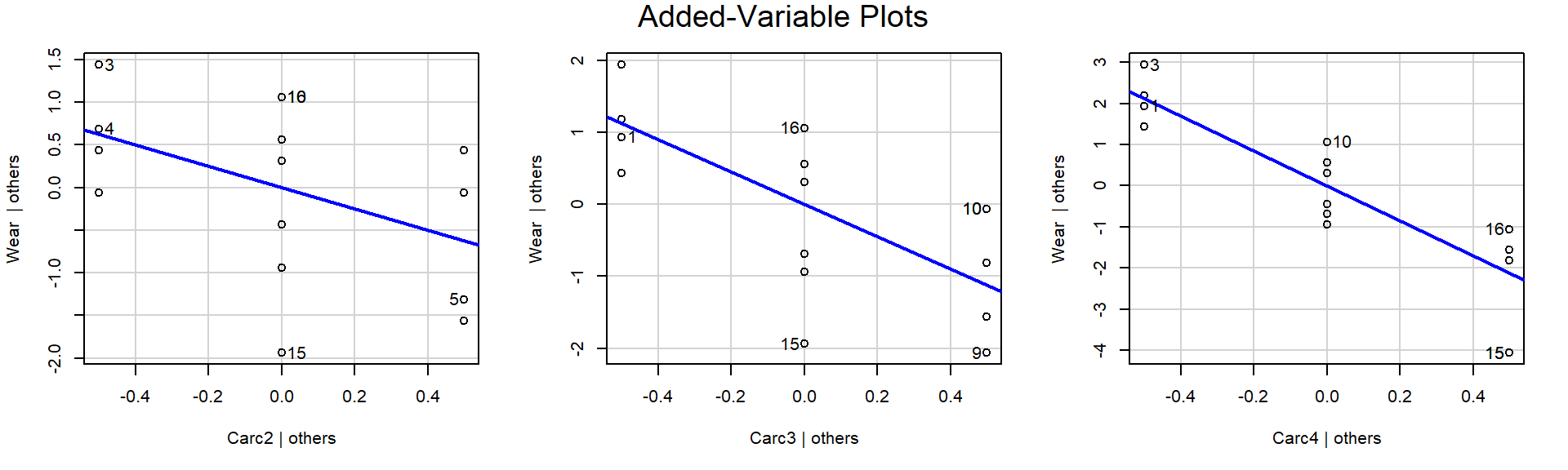Anderson-Darling normality test

data:  fit_d3\$residuals
A = 0.35174, p-value = 0.4226

## 4.4(1 p) Can we infer a difference in mean wear levels between the 4 brands?

It is appropriate to test whether there are differences between Brands controlling for the effect of Car. This is the additive model. Note that because the Car blocks are part of the experimental design, they should remain in the model regardless of whether the block is significant or not.

library(car)
Anova(fit_d3, type=3)
Anova Table (Type III tests)

Response: Wear
Sum Sq Df  F value    Pr(>F)
(Intercept) 598.94  1 466.2000 4.617e-09 ***
Brand        30.69  3   7.9622  0.006685 **
Car          38.69  3  10.0378  0.003133 **
Residuals    11.56  9
---
Signif. codes:  0 '***' 0.001 '**' 0.01 '*' 0.05 '.' 0.1 ' ' 1

## 4.5(2 p) Perform the pairwise comparisons and summarize numerically and graphically.

# Contrasts to perform pairwise comparisons
cont_d3_b <-
emmeans::emmeans(
fit_d3
, specs = "Brand"
)
# Means and CIs
cont_d3_b
 Brand emmean    SE df lower.CL upper.CL
A       14.2 0.567  9    12.97     15.5
B       12.2 0.567  9    10.97     13.5
C       10.8 0.567  9     9.47     12.0
D       11.0 0.567  9     9.72     12.3

Results are averaged over the levels of: Car
Confidence level used: 0.95 
# Pairwise comparisons
cont_d3_b %>% pairs()
 contrast estimate    SE df t.ratio p.value
A - B        2.00 0.801  9  2.495  0.1274
A - C        3.50 0.801  9  4.367  0.0080
A - D        3.25 0.801  9  4.055  0.0125
B - C        1.50 0.801  9  1.872  0.3041
B - D        1.25 0.801  9  1.560  0.4451
C - D       -0.25 0.801  9 -0.312  0.9888

Results are averaged over the levels of: Car
P value adjustment: tukey method for comparing a family of 4 estimates 

EMM plot interpretation

This EMM plot (Estimated Marginal Means, aka Least-Squares Means) is only available when conditioning on one variable. The blue bars are confidence intervals for the EMMs; don’t ever use confidence intervals for EMMs to perform comparisons – they can be very misleading. The red arrows are for the comparisons among means; the degree to which the “comparison arrows” overlap reflects as much as possible the significance of the comparison of the two estimates. If an arrow from one mean overlaps an arrow from another group, the difference is not significant, based on the adjust setting (which defaults to “tukey”).

# Plot means and contrasts
p <- plot(cont_d3_b, comparisons = TRUE)
p <- p + labs(title = "Tukey-adjusted Treatment contrasts")
p <- p + theme_bw()
print(p)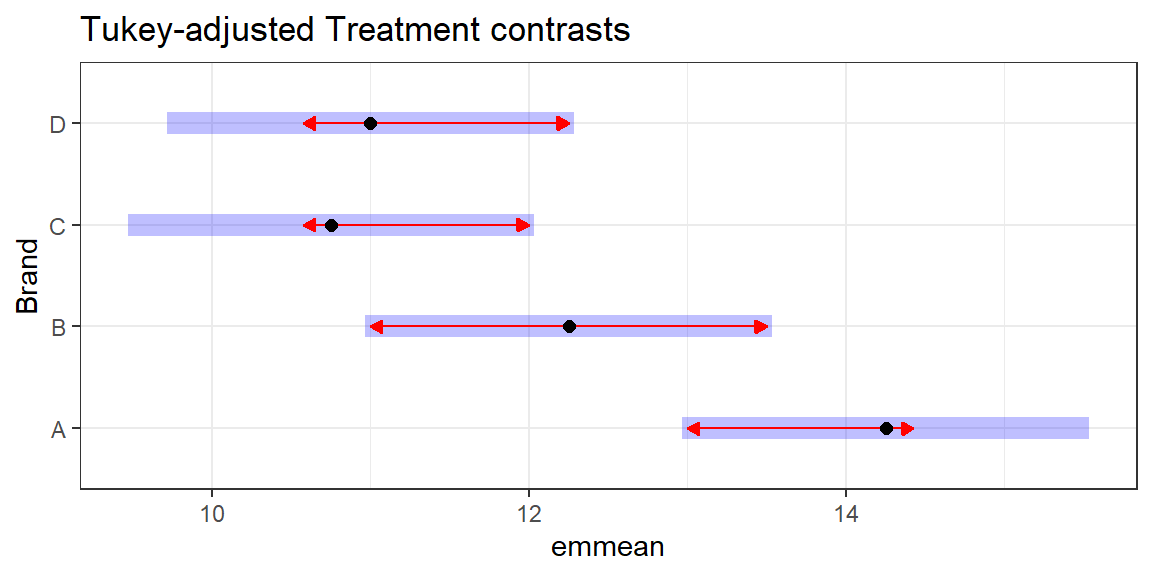Summarize the results in a table like this, where the effect of the Brands are sorted and the bars indicate pairs that are not statistically different. Then summarize in words.

Example:
Brand:     Z   Y   W   X
-------
-------